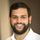cancel
Showing results for
Did you mean:Helper I

## Can't Solve Circular Dependency, CALCULATE Can't See Values

Hello!

I previously had a problem of replacing a value from a table three dependencies deep.  Below is the link and the solution I found.

I recently got another request for the same issue but to pull a different value.  Figured it wasn't a problem and just made a new column with the same code, replacing "Table4[Table 4 "account"]" with "Table4[Table 4 "new_request"]" (code below).

This caused the circular dependency error.  From my research, the solution is to add CALCULATE with a REMOVEFILTERS filter.  However, the code for some reason gives a "Cannot Find Name" error for all column names for table1, which is where the new column sits (third example below).

Moving the logic to a different table isn't a viable solution, and isn't sustainable anyway if these requests keep coming in.

Any help would be greatly appreciated, thank you!

(Original, no issues)

Replace =
IF('Table1'[Table 1 "names"] = "",
(
VAR Test1 = LOOKUPVALUE(Table2[Location 1 keys]Table2[Nameskey]Table1[Names keys])
VAR Test2 = LOOKUPVALUE(Table3[Location 2 keys]Table3[Location 1 keys]Test1)
VAR Test3 = LOOKUPVALUE(Table4[Table 4 "account"]Table4[Location 2 keys]Test2)
Return Test3
),
Table1[Table 1 "names"]
)

(New code, causes circular dependency error)

ReplaceNEW =
IF('Table1'[Table 1 "names"] = "",
(
VAR Test1 = LOOKUPVALUE(Table2[Location 1 keys]Table2[Nameskey]Table1[Names keys])
VAR Test2 = LOOKUPVALUE(Table3[Location 2 keys]Table3[Location 1 keys]Test1)
VAR Test3 = LOOKUPVALUE(Table4[Table 4 "new_request"]Table4[Location 2 keys]Test2)
Return Test3
),
Table1[Table 1 "names"]
)

(Attempted Solution, all Table1 columns give a "Cannot Find Name" error)

ReplaceNEW =
CALCULATE
(
IF('Table1'[Table 1 "names"]*ERROR* = "",
(
VAR Test1 = LOOKUPVALUE(Table2[Location 1 keys]Table2[Nameskey]Table1[Names keys]*ERROR*)
VAR Test2 = LOOKUPVALUE(Table3[Location 2 keys]Table3[Location 1 keys]Test1)
VAR Test3 = LOOKUPVALUE(Table4[Table 4 "new_request"]Table4[Location 2 keys]Test2)
Return Test3
),
Table1[Table 1 "names"]*ERROR*
),
REMOVEFILTERS(Table 1[Replace])
)

2 REPLIES 2Super User

Can you provide a screenshot of what your data model looks like and the tables you have? I think you just need to rework your model to avoid these confusing measures.Helper I

Here's the table layout:
Table Structure:
Table 1 (if blank) --> Table 2 --> Table 3 --> Table 4 (use this value)

Table 1 -> Table 2: Many to Many, Cross Filter Direction: Both
Table 2 -> Table 3: Many to One, Cross Filter Direction: Both
Table 3 -> Table 4: Many to One, Cross Filter Direction: Both

The data itself is imported from Dynamics 365 so I can't rework the model unfortunately.